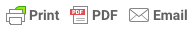## Basic Market Equilibrium Calculator

Confronted with any competitive market supply and demand situation, you want immediately to know the answers to four questions:

1. What is the equilibrium price at which the commodity is sold?
2. What is the equilibrium quantity sold?
3. What is the consumer surplus--how much is the existence of the market worth to buyers collectively?
4. What is the producer surplus--how much is the existence of the market worth to the sellers collectively?

If we are willing to assume linear demand and supply curves, we specify the behavior of demanders and suppliers with two parameters each:

• The maximum willingness-to-pay of the consumer who wants the commodity most.
• The number of additional units bought for each $1 drop in the price. And: • The minimum willingness-to-supply of the producer who can make the commodity cheapest. • The number of additional units sold for each$1 increase in the price.

Specify those four numbers under "basic conditions" below, click the "Solve the Model" button, and you will know what you need to know...

### The Basic Conditions: Supply and Demand

• <-- Maximum willingness to pay
• <-- Additional customer demand from cutting price by $1 • <-- Minimum willingness to supply • <-- Additional producer supply from raising the price by$1

### The Market Equilibrium

• the price <-- The equilibrium price
• the quantity <-- The equilibrium quantity
• the consumer surplus <-- The equilibrium consumer surplus
• the producer surplus <-- The equilibrium producer surplus